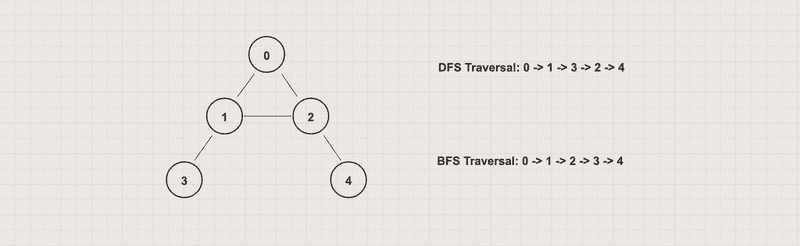# HelloKoding

Practical coding guides

# Depth First Search Algorithms in Graph with Java Examples

In this article, you will learn to implement Depth First Search (DFS) algorithms in a graph by using Java with iterative and recursive approaches

Depth First Search (DFS) is an algorithm for traversing or searching for a graph. The algorithm starts at an arbitrary node and explores as far as possible along each branch before backtracking

Let’s get started!

## Problem

• Give an undirected/directed graph G(V, E)
• Write a Depth-First search algorithm to print out each vertex value exactly once

## ExampleFor the above graph with 0 as the starting vertex, assuming that the left edges are chosen before the right edges, the DFS traversal order will be 0 -> 1 -> 3 -> 2 -> 4

## Approach 1: Iterative

• Use an array to track visited nodes to avoid processing a node more than once
• Use a stack to track which nodes to visit next

DFSByIterative.java

``````package com.hellokoding.datastructure.graph;

import java.util.ArrayDeque;
import java.util.Deque;

public class DFSByIterative {
static void dfsByIterative(GraphUndirectedByAdjacencyList g, int v) {
boolean[] visited = new boolean[g.getV()];

Deque<Integer> stack = new ArrayDeque<>();
stack.push(v);

while (!stack.isEmpty()) {
v = stack.pop();

if (!visited[v]) {
visited[v] = true;
System.out.printf("%d ", v);

stack.push(w);
}
}
}
}

public static void main(String[] args) {

dfsByIterative(g, 0);
}
}
``````

`GraphUndirectedByAdjacencyList` is defined in Graph Data Structure

• Output
``0 1 3 2 4 ``
• Time complexity: O(V+E)
• Space complexity: O(V)

## Approach 2: Iterative with Color

• Use a color array to track vertex states. Each vertex can have 3 states marked by color
• White represents unvisited
• Gray represents a visit in progress
• Black represents visited
• Use a stack to track which nodes to visit next

DFSByIterativeWithColor.java

``````package com.hellokoding.datastructure.graph;

import java.util.ArrayDeque;
import java.util.Deque;

public class DFSByIterativeWithColor {
static final int WHITE = 0, GRAY = 1, BLACK = 2;

static void dfsByIterativeWithColor(GraphUndirectedByAdjacencyList g, int v) {
int[] color = new int[g.getV()];

Deque<Integer> stack = new ArrayDeque<>();
stack.push(v);

while (!stack.isEmpty()) {
v = stack.pop();

if (color[v] == WHITE) {
color[v] = GRAY;
System.out.printf("%d ", v);

stack.push(w);
}

color[v] = BLACK;
}
}
}

public static void main(String[] args) {

dfsByIterativeWithColor(g, 0);
}
}
``````

`GraphUndirectedByAdjacencyList` is defined in Graph Data Structure

• Output
``0 1 3 2 4 ``
• Time complexity: O(V+E)
• Space complexity: O(V)

## Approach 3: Recursive

• Use an array to track visited nodes to avoid processing a node more than once
• Instead of using a stack, the DFS algorithm calls to itself to explore unvisited vertices

DFSByRecursive.java

``````package com.hellokoding.datastructure.graph;

public class DFSByRecursive {

static void dfs(GraphUndirectedByAdjacencyList g, int v, boolean[] visited) {
visited[v] = true;
System.out.printf("%d ", v);

for (Integer w : g.getAdjacencyList().get(v)) {
if (!visited[w]) {
dfs(g, w, visited);
}
}
}

boolean[] visited = new boolean[g.getV()];

for (int i = 0; i < g.getV(); i++) {
if (!visited[i]) {
dfs(g, i, visited);
}
}
}

public static void main(String[] args) {

traversal(g);
}
}
``````

`GraphUndirectedByAdjacencyList` is defined in Graph Data Structure

• Output
``0 2 1 3 4 ``
• Time complexity: O(V+E)
• Space complexity: O(V)

## Approach 4: Recursive with Color

• Use a color array to track vertex states. Each vertex can have 3 states marked by color
• White represents unvisited
• Gray represents a visit in progress
• Black represents visited
• Instead of using a stack, the DFS algorithm calls to itself to explore White vertices

DFSByRecursiveWithColor.java

``````package com.hellokoding.datastructure.graph;

public class DFSByRecursiveWithColor {
static final int WHITE = 0, GRAY = 1, BLACK = 2;

static void dfsByRecursiveWithColor(GraphUndirectedByAdjacencyList g, int v, int[] color) {
color[v] = GRAY;
System.out.printf("%d ", v);

for (Integer w : g.getAdjacencyList().get(v)) {
if (color[w] == WHITE) {
dfsByRecursiveWithColor(g, w, color);
}
}
color[v] = BLACK;
}

int[] color = new int[g.getV()];

for (int i = 0; i < g.getV(); i++) {
if (color[i] == WHITE) {
dfsByRecursiveWithColor(g, i, color);
}
}
}

public static void main(String[] args) {

traversal(g);
}
}
``````

`GraphUndirectedByAdjacencyList` is defined in Graph Data Structure

• Output
``0 2 1 3 4 ``
• Time complexity: O(V+E)
• Space complexity: O(V)

## Applications

• Detect Cycle in a Directed Graph

You can use DFS to detect a cycle in a directed graph. When visiting a vertex v and its adjacency vertices, if there is a back edge (w, v) which directs to v then that graph has a cycle